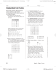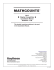## Transcription

```AwesomeMath Admission Test Cover Sheet
Last Name
Contact Information
First Name
A
Phone Number
Email
Number of pages (not including this cover sheet)
B
C
Check one
Awesome Math Test A
March 16 - April 6, 2012
• Do not be discouraged if you cannot solve all of the questions: the test is not
made to be easy. We want to see the solutions you come up with no matter how
many problems you solve.
• Include all significant steps in your reasoning and computation. We are
receive much less credit than well-reasoned progress towards a solution without
• In this document, you will find a cover sheet and an answer sheet. Print out
each one and make several copies of the blank answer sheet. Fill out the top of
each answer sheet as you go, and then fill out the cover sheet when you are
finished. Start each problem on a new answer sheet.
• All the work you present must be your own.
• Do not be intimidated! Some of the problems involve complex mathematical
ideas, but all can be solved using only elementary techniques, admittedly
combined in clever ways.
• Be patient and persistent. Learning comes more from struggling with problems
than from solving them. Problem-solving becomes easier with experience.
Success is not a function of cleverness alone.
• Make sure that the cover sheet is the first page of your submission, and that it is
completely filled out. Solutions are to be emailed to [email protected] or
Dr. Titu Andreescu
3425 Neiman Road, Plano TX 75025
E-mailed solutions may be written and scanned or typed in LaTeX. They should
be sent as an attachment in either .doc or .pdf format. If you write and scan your
solutions, insert the scans into a .doc or .pdf file and send just the one file.
Please go to the next page for the problems
Test B
March 16 — April 6
1. The sum of some consecutive integers is 2012. Find the smallest of
these integers.
2. How many 5-digit numbers have at least one digit of 5?
3. Find all integers n for which n2 − n + 1 divides n2012 + n + 2001.
4. Let a and b be real numbers such that 2a2 + 3ab + 2b2 ≤ 7. Prove that
max(2a + b, a + 2b) ≤ 4.
5. Find all pairs (m, n) of integers such that m3 + n3 = 2015.
6. Let P (x) = 3x3 − 9x2 + 9x. Prove that P (a2 + b2 + c2 ) ≥ P (ab + bc + ca)
for all real numbers a, b, c.
7. For a positive integer N , let r(N ) be the number obtained by reversing
the digits of N . For example, r(2013) = 3102. Find all 3-digit numbers
N for which r2 (N ) − N 2 is the cube of a positive integer.
8. Solve the system of equations
8
9
5
, log yz =
, log zx =
.
log xy =
log z
log x
log y
9. In circle C chords AB and XY intersect at P . Prove that the projections of P onto AX, BX, AY , BY are concyclic if and only if the
midpoints of AX, BX, AY , BY are the vertices of a rectangle.
10. (a) Give example of a triple (a, b, c) of even positive integers such
that ab + 1, bc + 1, ca + 1 are all perfect squares.
(b) Are there triples (a, b, c) of odd positive integers such that ab + 1,
bc + 1, ca + 1 are all perfect squares?
Problem Number
Page
Of
Write neatly! All work should be inside the box. Do NOT write on the back of the page!
```

#### Similar documents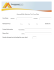### Discrete Math, Spring 2013 - Some Sample Problems### File - 6th Grade Math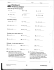### Homework 3### Sample Individual Questions 2012 Canadian Team Mathematics Contest (CTMC)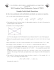### Study guide 7.3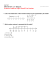### Document 6487473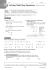### The Paperless Math Classroom Brian Aspinall Teacher, LKDSB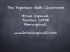### Sample Problems Algebra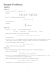### holiday homework navy children school 2015-2016 class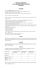### Sample Final Exam Questions (Mandatory Part)### HOW TO COMPLETE THE ANSWER SHEET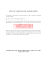### PROBLEM SET 01 - Proofs and induction 1 Proofs 2 Induction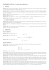### Exercises 09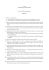### MPM1D Unit 3 - Mr. Murray Teaches Math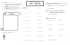### CSE5473 Homework 1 (due Wednesday, February 11) 0. A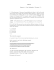### Notes on algorithms, and examples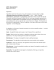### Standardized Test Practice